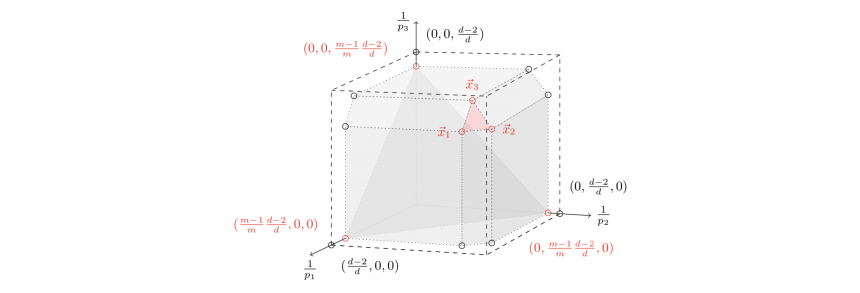# AnalysisPermanent faculty and their fields of interest

Edward AzoffProfessorPh.D. University of Chicago, 1972. Interface of operator theory and descriptive set theory.

Joseph H.G. Fu, ProfessorPh.D. Massachusetts Institute of Technology, 1984. "Integral geometric regularity”, the study of the conditions under which classical curvature integrals may be extracted from spaces with singularities.

Neil LyallProfessorPh.D. University of Wisconsin, 2004. Arithmetic combinatorics and harmonic analysis.

Akos MagyarProfessor, Ph.D. Princeton, 1996. Discrete harmonic analysis. Arithmetic combinatorics. Analytic methods for diophantine problems.

Jingzhi TieProfessorPh.D. University of Toronto, 1995. Partial differential equations, several complex variables, and financial mathematics.

Shuzhou Wang, Associate ProfessorPh.D. University of California, Berkeley, 1993. Quantum Groups, Non-commutative Geometry, Operator Algebras, and Mathematical Physics.

Postdoctoral associates and their fields of interest

Jeremy SchwendPostdoctoral Research and Teaching Associate, Ph.D. University of Wisconsin, 2020. Harmonic analysis, encompassing averaging operators, restriction, and decoupling, with a focus on Euclidean harmonic analysis and problems related to curvature.

Zhenhua Wang, Limited Term Assistant Professor, Ph.D. University of Houston, 2019. Operator algebra theory, and more specifically in the following areas: Operator algebras, Jordan operator algebras, operator spaces, and operator theory.

Hans D. Parshall (Neil Lyall/Akos Magyar), Point configurations over finite fields, 2017.

Lauren E. Huckaba (Neil Lyall), Simplices and sets of positive upper density in R^d, 2016.

Phong Luu (Qing Zhang/Jingzhi Tie), Optimal Pairs Trading Rules and Numerical Methods, 2016.

Due Nguyen (Qing Zhang/Jingzhi Tie), Optimal asset trading under regime switching models, 2013.

Alex Rice (Neil Lyall), Improvements and extensions of two theorems of Sarkozy, 2012.

Jim Blair (Akos Magyar), On the embedding of triangles into integer lattices, 2004.

## Research Areas

Additive Combinatorics and Discrete Analysis Research Group at the Department of Mathematics at the University of Georgia.

## Algebra

The algebra group has a vibrant training program for graduate students and postdoctoral fellows.
The algebra group has sponsored three NSF Postdoctoral Fellows: Wayne Wheeler (1996-98), David Hemmer (2001-03) and Jonathan Kujawa (2003-07). If you interested in applying to the Department of Mathematics at UGA for graduate studies or a postdoc, please do not hestitate to contact one of our faculty members.

## Algebraic Geometry

Some focus areas of the Algebraic Geometry Research Group are moduli spaces, minimal model program, K3 surfaces and abelian varieties, combinatorial algebraic geometry. The group members also share a wide circle of interests with connections to arithmetic geometry, symplectic geometry and toplogy, combinatorics, and representation theory.

## Applied Mathematics

The Applied Mathematics Group in the Department of Mathematics at the University of Georgia has scientific interests in Approximation Theory, Computational Math and High Performance Computing, Control Theory and PDE control, Dynamical Systems, Mathematical Biology, Mathematical Ecology, Mathematical Finance, Machine Learning, Numerical Solutions to PDEs, Signal, Image and Video Processing, Sparse Solutions of Linear System, Energy Minimization Problems and Mathematical Models for Collective Behavior. The Applied Math group members are currently investigating various topics including stability in population dynamics models, network analysis, microbial community analysis, compressive sensing, deep learning for data approximation, stochastic filtering, multivariate spline methods for various applications, numerical methods for kinetic equations, existence/uniqueness/stability of energy minimizers and long time behavior of models for collective behavior.

## Geometry

The interests of the Geometry research area group at the Department of Mathematics at the University of Georgia revolve around differential geometry, broadly construed. We have a weekly seminar that meets on Fridays at 2:30.

## Topology

Each summer since 1961 we have hosted the Georgia Topology conference and every eighth year it's "the big one", a 2-week long festival of topology which we call the Georgia International Topology Conference.  For additional information see our conference webpage.